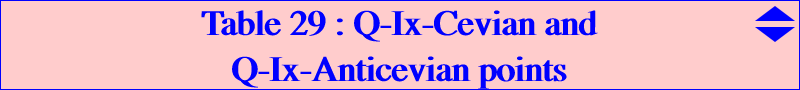Let Q be a fixed point. A point P is said to be a Q-Ix-cevian (or anticevian) point if Q is an in/excenter of the cevian (or anticevian) triangle PaPbPc of P. Q-Ix Cevian points When Q = H, the cevian triangle PaPbPc is the orthic triangle and its in/excenters are H, A, B, C. Hence H is a H-Ix-cevian point or more simply an Ix-cevian point. Compare with Table 23 : Ix-anticevian points. We now suppose that Q is not H and we seek such Q-Ix-cevian points.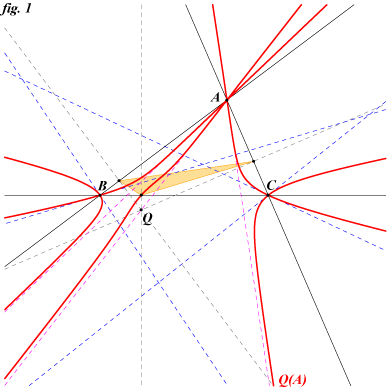Let P be a point and PaPbPc its cevian triangle. When we express that Q lies on a bisector at Pa in the triangle PaPbPc we find that P must lie on a circum-quintic denoted by Q(A). Q(A) has a triple point at A and one of the tangents is the line AQ. The two other tangents are not necessarily real. B and C are nodes and the four (not necessarily real) tangents at these points form a complete quadrilateral whose vertices are the fixed points of an isoconjugation. Q(A) also contains the A-vertex of the pedal triangle of Q which is the "last" point on BC. See figure1.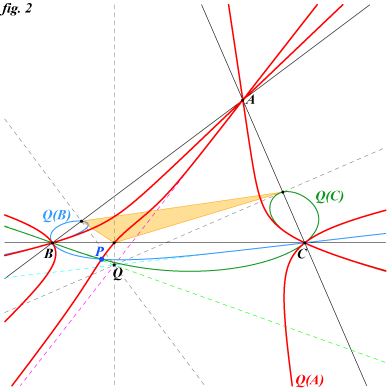Similarly, we define the two other quintics Q(B) and Q(C). Naturally, P is a Q-Ix-cevian point if and only if it lies on two of these quintics and then obviously on the third one. Q(A), Q(B) and Q(C) generate a net of quintics passing through P and each quintic has the form Q(M) = l Q(A) + m Q(B) + n Q(C) where M = l:m:n is any point of the plane. See figure 2.When we choose M = G, we find that Q(M) decomposes into the sidelines of ABC and the rectangular circum-hyperbola H(Q) passing through Q. Hence, the sought points P must lie on H(Q) and on any of the quintics. H(Q) and Q(A) meet at ten points, A (thrice), B(twice), C(twice) and three other points which are the requested points P. One must be real and the remaining two can be real or imaginary. Thus, For any point Q which is not H, there is always one real Q-Ix-cevian point P. See figure 3.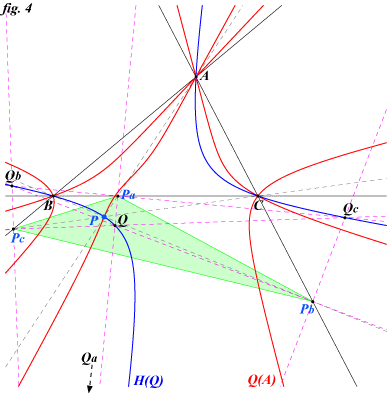Another explanation is the following. The rectangular hyperbola passing through P and the four in/excenters of PaPbPc is a diagonal conic with respect to PaPbPc. It must contain the vertices of the anticevian triangle (with respect to PaPbPc)  of any of its point and in particular it contains A, B, C. It follows that the points which have an in/excenter of their cevian triangle at Q must lie on the rectangular circum- hyperbola passing through Q. Hence, H(Q) contains the in/excenter Q and also the three other in/excenters Qa, Qb, Qc. See figure 4.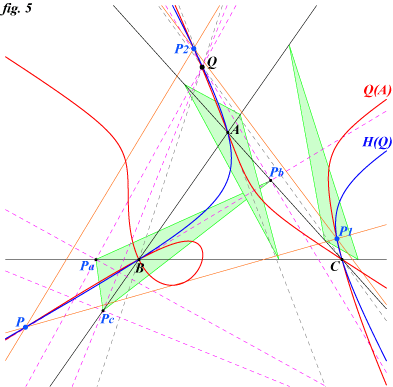In figure 5, one can see three real Q-Ix-cevian points P, P1, P2 obtained with Q homothetic of H under h(O,3).Q-Ix Anticevian points Let Q be a point, XaXbXc and QaQbQc its anticevian and pedal triangles respectively. We seek P such that the anticevian triangle PaPbPc of P has an in/excenter at Q in which case P is said to be a Q-Ix-anticevian point.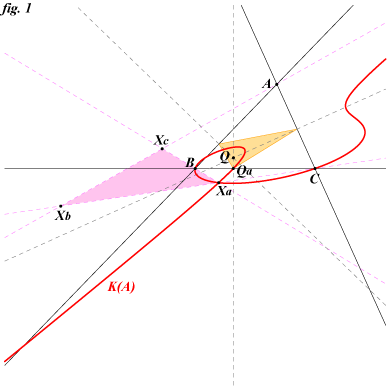When we write that a bisector at Pa in the triangle PaPbPc passes through Q, we find a cubic K(A) passing through B, C, Qa and Xa which is a node on the cubic. K(A) contains A if and only if Q lies on a bisector at A in the triangle ABC. See figure 1. Similarly, the cubics K(B) and K(C) are defined. Naturally, P is a Q-Ix-anticevian point if and only if it lies on two of these cubics and then obviously on the third one. K(B) and K(C) meet at A and two other (not always real) points A1, A2 on the sideline BC. Hence, they must have six other common points - also on K(A) - which cannot lie on a sideline of ABC. For any point Q, there are at most six Q-Ix-anticevian points P. K(A), K(B) and K(C) generate a net of cubics passing through these six points and each cubic has the form K(M) = l K(A) + m K(B) + n K(C) where M = l:m:n is any point of the plane. The figure 2 shows an example with two real Q-Ix-anticevian points.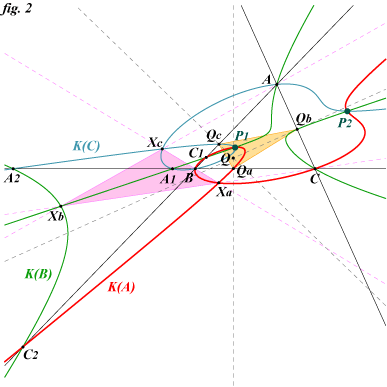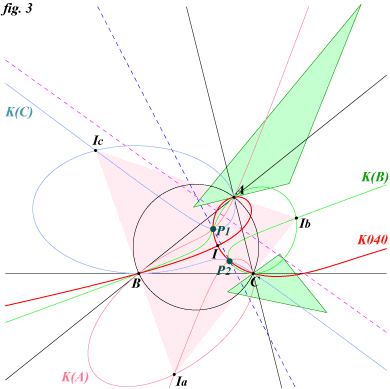A special case Note that the three cubics are circum-cubics if and only if Q is an in/excenter of ABC. In this case, K(A), K(B) and K(C) belong to a same pencil of circum-cubics. With Q = I = X(1) (incenter), the pencil contains the Pelletier strophoid K040 which must pass through the six I-Ix-anticevian points. The figure 3 shows a triangle ABC for which there are two real I-Ix-anticevian points with their corresponding anticevian triangles.When we choose N = p(2 SA qr + b^2r^2 + c^2q^2) : : , K(N) is a cubic passing through the vertices Xa, Xb, Xc of the anticevian triangle of Q. Moreover, the cubic meets the sidelines of XaXbXc again at three collinear points Ya, Yb, Yc. The trilinear pole (with respect to XaXbXc) of the line L(Q) through these points is the point R = p^3(cq-br)(cq+br) : : , the tangents at Xa, Xb, Xc to the cubic form a triangle ZaZbZc perspective with XaXbXc with a rather complicated perspector R*. In fact, this cubic is a nK0 with respect to XaXbXc whose root is R. See figure 4 below.Let now f be the homology with center Q, with axis the trilinear polar of Q (with respect to ABC) which transforms Xa, Xb, Xc into A, B, C respectively. f is given by u:v:w -> p^2(rv + qw) : : and its reciprocal transformation g by u:v:w -> p(-u/p + v/q + w/r) : : . This homology f transforms K(N) into a nK0 (in the triangle ABC) denoted by K'(N) whose pole is W = p^4(2 SA qr + b^2r^2 + c^2q^2) : : , whose root is R as seen above. One remarkable thing to observe is that these cubics K(N) and K'(N) contain Q if and only if Q lies on the Euler-Morley quintic Q003. This was the case when Q = I with the Pelletier strophoid K040.